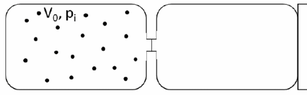# Finding the increase in entropy of the universe in gas expansion

• lorenz0
In summary, the conversation discusses the correct equations for calculating pressure and heat in a gas system, as well as the incorrect equation for calculating the entropy of the universe. It is found that the entropy change of the universe is the sum of the entropy change of the system and the entropy change of its surroundings.

#### lorenz0

Homework Statement
Two boxes of equal volume ##V_0## are separated by a membrane. The first one to the left, thermally isolated contains ##n## moles of perfect biatomic gas at pressure ##p_i## and temperature ##T_i##. The second box is empty, and in contact with a source of heat at a temperature which is ##T_i/2##. At a certain instant the membrane is removed and the gas reaches a new equilibrium. Find: a) the final pressure of the gas ##P_f## b) the heat ##Q## exchanged with the source of heat c) the variation of entropy of the universe ##\Delta U_{univ}##
Relevant Equations
##PV=nRT, \Delta U=nC_V \Delta T=Q-L, \Delta S=nC_V \ln(\frac{T_f}{T_i})+nR\ln(\frac{V_f}{V_i})##
a) ##P_f=\frac{nRT_f}{V_f}=\frac{nR\frac{T_i}{2}}{2V_0}=\frac{1}{4}\frac{nRT_i}{V_0}=\frac{1}{4}P_i##

b) ##Q=\Delta U=nC_V \Delta T=n\frac{5}{2}R(-\frac{T_i}{2})=-\frac{5}{4}nRT_i=-\frac{5}{4}P_i V_0## (##L=0## since the gas expands in a vacuum;

Now, (a) and (b) are both correct but not (c), for which I get:

c) ##\Delta S_{system}=nC_V \ln(\frac{T_f}{T_i})+nR\ln(\frac{V_f}{V_i})=n\frac{5}{2}R\ln(\frac{\frac{T_i}{2}}{T_i})+nR\ln(\frac{2V_0}{V_0})=-\frac{3}{2}nR\ln(2)## I understand that the entropy of the system decreases since heat goes out of the system so I guessed that the entropy of the universe should go up by the same amount but apparently this is wrong as in the solution given in the text is ##n\frac{5}{2}R-\frac{3}{2}nR\ln(2)##. What is it that I am missing in finding the entropy of the universe? How should I reason about such a problem in general? Thanks

#### Attachments

•Joule_expansion.png
13.3 KB · Views: 70
The surroundings is being treated as an ideal constant temperature reservoir at temperature ##T_i/2##. The amount of heat it receives at this temperature is ##+\frac{5}{4}nRT_i##. So what is its entropy change?

Otherwise, you did a great job of analyzing this.

Chestermiller said:
The surroundings is being treated as an ideal constant temperature reservoir at temperature ##T_i/2##. The amount of heat it receives at this temperature is ##+\frac{5}{4}nRT_i##. So what is its entropy change?
It is ##\frac{Q}{T_i /2}=\frac{\frac{5}{4}nRT_i}{\frac{T_i}{2}}=\frac{2}{T_i}\frac{5}{4}nRT_i=\frac{5}{2}nR## so ##\Delta S_{universe}=\frac{5}{2}nR-\frac{3}{2}nR\ln(2)=\frac{nR}{2}(5-3\ln(2))##. So the entropy change of the universe is the sum of the entropy change of the system + entropy change of its surroundings, right?

•Chestermiller
lorenz0 said:
It is ##\frac{Q}{T_i /2}=\frac{\frac{5}{4}nRT_i}{\frac{T_i}{2}}=\frac{2}{T_i}\frac{5}{4}nRT_i=\frac{5}{2}nR## so ##\Delta S_{universe}=\frac{5}{2}nR-\frac{3}{2}nR\ln(2)=\frac{nR}{2}(5-3\ln(2))##. So the entropy change of the universe is the sum of the entropy change of the system + entropy change of its surroundings, right?
Yes. Very nice.

Chestermiller said:
Yes. Very nice.
You have been very helpful: thanks!